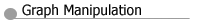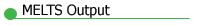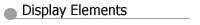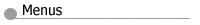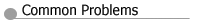# Disk output from MELTS

Disk output from MELTS comes in two varieties. A comprehensive output file named melts.out records a continuous record of the evolution of the system at every stage in a model calculation. If MELTS version 1.1.3 or greater is running, then the results written to melts.out are also neatly formatted into one or more tabular output files named melts-liquid.tbl and solid phase name..tbl

## Contents of the file melts.out

Here is an example of a typical entry in the melts.out file. Click on an item of interest to get a description.

```**********----------**********
Title: Equilibrium Crystallization of MORB

T = 1185.00 (C)  P = 0.500 (kbars)  log(10) f O2 = -9.46  delta HM = -6.58  NNO = -1.72  QFM = -0.98  IW = 2.48

Constraint Flags:

Liquid           mass = 67.31 (gm)  density = 2.68 (gm/cc)  viscosity = 2.24 (log 10 poise)     (analysis in wt %)
G = -1080481.67 (J)  H = -816869.52 (J)  S = 180.79 (J/K)  V = 25.08 (cc)  Cp = 99.28 (J/K)
SiO2   TiO2  Al2O3  Fe2O3  Cr2O3    FeO    MnO    MgO    NiO    CoO    CaO   Na2O    K2O   P2O5    H2O
49.19   1.48  14.92   1.25   0.04   9.39   0.00   8.11   0.00   0.00  12.10   3.05   0.04   0.12   0.30

olivine          mass = 7.01 (gm)  density = 3.27 (gm/cc)     (analysis in mole %)
(Ca0.01Fe''0.17Mg0.83)2SiO4
G = -110763.65 (J)  H = -86081.59 (J)  S = 16.93 (J/K)  V< /A> = 2.14 (cc)  Cp = 8.71 (J/K)
fayalite    forsterite  monticellite
16.66         81.87          1.47

pyroxene         mass = 3.37 (gm)  density = 3.21 (gm/cc)     (analysis in mole %)
cpx Na0.01Ca0.82Fe''0.14Mg0.90Fe'''0.04Ti0.01Al0.20Si1.88O6
G = -55066.68 (J)  H = -43558.83 (J)  S = 7.89 (J/K)  V = 1.05 (cc)  Cp = 4.02 (J/K)
diopside clinoenstatit  hedenbergite alumino-buffo    buffonite       esseneite      jadeite
55.26         16.68         14.46          8.65         -6.04          9.75          1.24

feldspar         mass = 22.66 (gm)  density = 2.67 (gm/cc)     (analysis in mole %)
K0.00Na0.23Ca0.77Al1.77Si2.23O8
G = -393422.55 (J)  H = -312917.95 (J)  S = 55.21 (J/K)  V = 8.50 (cc)  Cp = 27.91 (J/K)
albite     anorthite      sanidine
23.02         76.95          0.03

Total solids     mass = 33.04 (gm)  density = 2.83 (gm/cc)
G = -559252.88 (J)  H = -442558.38 (J)  S = 80.03 (J/K)  V = 11.69 (cc)  Cp = 40.64 (J/K)

Viscosity of the System: 3.12 (log 10 poise)

System           mass = 100.35 (gm)  density = 2.73 (gm/cc)
G = -1639734.54 (J)  H = -1259427.90 (J)  S = 260.81 (J/K)  V = 36.77 (cc)  Cp = 139.91 (J/K)```

The *.tbl files are formatted as fields separated by commas. Each record ends with a carriage return-linefeed character. One record is produced for every equilibrium state of the system reported in melts.out.

## Contents of the file melts-liquid.tbl

The file melts-liquid.tbl is always created when MELTS version 1.1.3 or greater is run. The first record contains the following field labels, delimited by commas:

• T (C) - Temperature of the system (Centigrade)
• P (kbars) - Pressure of the system (kilobars)
• log(10) f O2 - Base 10 logarithm of the oxygen fugacity
• liq mass (gm) - Current mass of the liquid phase
• liq rho (gm/cc) - Current density of the liquid phase
• liq vis (log 10 poise) - Base 10 logarithm of the liquid viscosity
• liq G (kJ), liq H (kJ), liq S (J/K), liq V (cc), liq Cp (J/K) - Liquid thermodynamic properties (extensive basis)
• wt% SiO2, wt% TiO2, wt% Al2O3, wt% Fe2O3, wt% Cr2O3
• wt% FeO, wt% MnO, wt% MgO, wt% NiO, wt% CoO
• wt% CaO, wt% Na2O, wt% K2O, wt% P2O5, wt% H2O - Liquid composition in wt% oxides
• sol mass (gm) - Current total mass of the solid phases in the system
• sol rho (gm/cc) - Current proportionally averaged density of the solid phases in the system
• sol G (kJ), sol H (kJ), sol S (J/K), sol V (cc), sol Cp (J/K) - Proportionally averaged solid thermodynamic properties (extensive basis)

Subsequent records contain numerical values corresponding to these quantities. melts-liquid.tbl may be read by standard graphics software that can import data tables with comma delimited fields.

## Contents of the file mineral.tbl

The remaining *.tbl files (output by MELTS version 1.1.3 or greater) contain information specific to mineral phases crystallizing from the magma. File names correspond to the mineral name with the .tbl extension. The files are created only if the mineral appears in the system. Records are written only if the mineral is crystallizing. When more than one composition of the same phase is crystallizing simultaneously (i.e. two feldspars or three pyroxenes), multiple r ecords are written containing the coexisting phase information.

The first record contains the following field labels, delimited by commas:

• T (C) - Temperature of the system (Centigrade)
• P (kbars) - Pressure of the system (kilobars)
• log(10) f O2 - Base 10 logarithm of the oxygen fugacity
• mass (gm) - Current mass of the solid phase
• rho (gm/cc) - Current density of the solid phase
• G (kJ), H (kJ), S (J/K), V (cc), Cp (J/K) - Solid phase thermodynamic properties (extensive basis)
• Component #1, Component #2, ... - Current solid phase composition in terms of mole fractions of the indicated thermodynamic components

Subsequent records contain numerical values corresponding to these quantities. The *.tbl files may be read by standard graphics software that can import data tables with comma delimited fields.

Back to Top